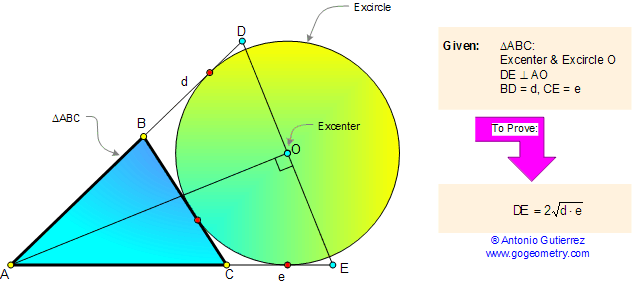Problem 66. Triangle, Excircle, Tangents, Geometric Mean. Level: High School, SAT Prep, College

 < PREVIOUS PROBLEM  |  NEXT PROBLEM > In a triangle ABC, O is the excenter, DE is perpendicular to AO. If BD = d and CE = e, prove that DE is the geometric mean of d and e that is $$DE = 2\sqrt{d \cdot e}$$.Tangents to an Excircle from the Wolfram Demonstrations Project by Jay Warendorff
 Home | Geometry | Search | Problems | Problems 61-70 | Geometric Mean | Wolfram Demonstrations Project | Email | by Antonio Gutierrez Post or view a solution to the problem 66